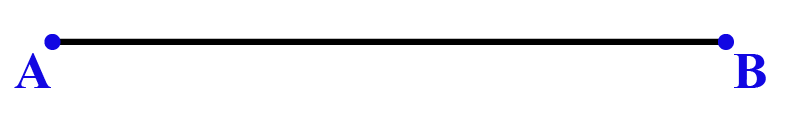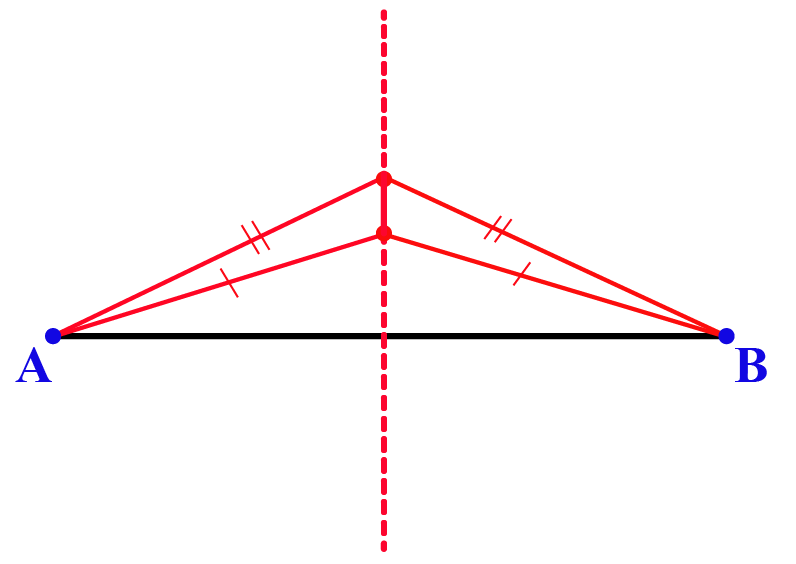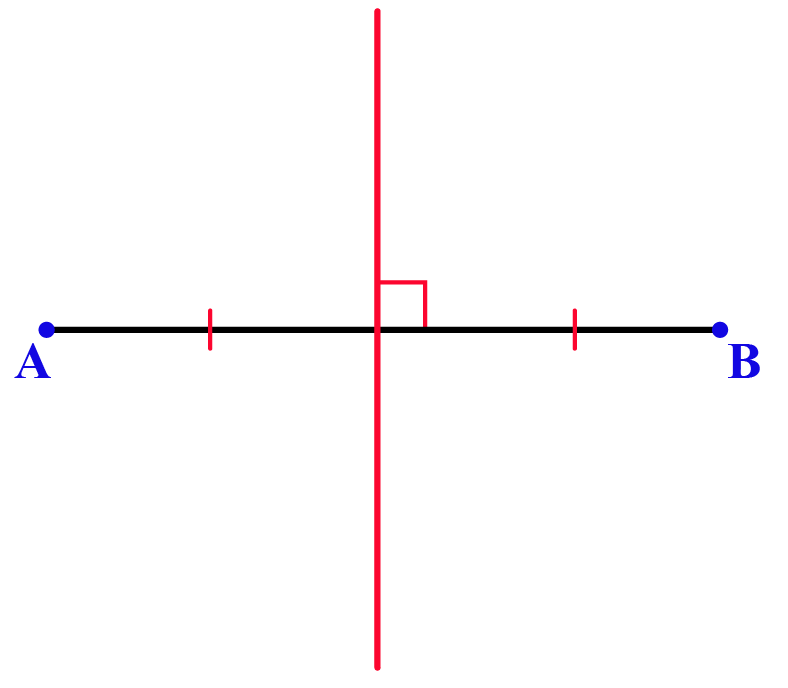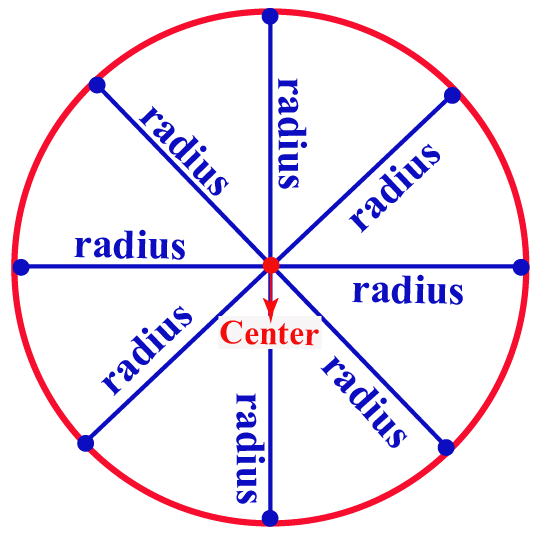# Locus

Locus

In this mini-lesson, we are going to learn about the definition of locus along with some examples of locus.

We are aware of the fact that earth revolves around the sun in an elliptical orbit.

This elliptical orbit is formed by joining the positions of the earth at different instances.

You can see this using the following illustration.

The curve joining all the positions of the earth is called the locus in this case.

EVery locus (curve) is associated with an equation which is called the equation of locus.

The curve and its equation depend upon the given locus problem.

Do we have a locus formula to find its equation? Let's see.

## Lesson Plan

 1 What is Meant by Locus? 2 Important Notes on Locus 3 Solved Examples on Locus 4 Interactive Questions on Locus 5 Challenging Questions on Locus

## What is Meant by Locus?

### Definition of Locus

"Locus" basically means a shape or a curve.

We know that any shape or curve is formed by a set of points.

So "Locus" is a set of points.

In math, the locus is a set of points that satisfy a set of rules.

## What is Locus of Points?

The locus of points is a curve or a line in two-dimensional geometry.

### Example

Consider a line segment $$\overline{AB}$$.Let us find the locus of all the points that are equidistant from A and B.

Let us place all points where each point is equidistant from A and B.Join all such points by a line. The resultant line is the perpendicular bisector of $$\overline{AB}$$.Thus, the locus of all points that are equidistant from A and B is the perpendicular bisector of $$\overline{AB}$$.

## What is Locus of Circle?

The locus of the set of all points that are at a fixed distance from a fixed point is called a circle.

Here,

• The fixed-point is called the "center" of the circle
• The fixed-distance is called the "radius" of the circleNot only the points marked in blue, there are an infinite number of points on the circle (locus).

Some other examples of locus are mentioned in the following "Important Notes."

## What are Locus Points in Two-Dimensional Geometry?

Locus points are the points on the curve (or) line that is associated with the locus.

Every locus is associated with an equation and let's see how to find it.

### Locus Formula

There is no specific formula to find the locus.

Here are the steps to find the locus of points in two-dimensional geometry,

• Assume any random point $$P(x,y)$$ on the locus.
• Write an equation depending on the given condition.
• Simplify it to get the equation of the locus.

### Locus Problem

Find the locus of a point that is at a distance of 4 units from a point $$(-3, 2)$$ in the xy-plane.

Solution

Let us assume that $$P(x,y)$$ is a point on the locus.

Then by the given condition,

The distance between $$(x,y)$$ and $$(-3, 2)$$ is 4

\begin{aligned} \sqrt{(x-(-3))^2+(y-2)^2}&=4\\[0.2cm] \text{Squaring on both sides},\\[0.2cm] (x+3)^2+(y-2)^2&=16\\[0.2cm] x^2+6x+9+y^2-4y+4&=16\\[0.2cm] x^2+y^2+6x-4y-3&=0 \end{aligned}

Thus, the locus of the required points is, $x^2+y^2+6x-4y-3=0$

## Solved Examples on Locus

Here are some more examples of locus.

 Example 1

Can we help Ava find the equation of locus of a point which is equidistant from the points $$A(-2, 0)$$ and $$B(3, 2)$$?

What does the equation of locus in this case represent?

Solution

Let us assume that $$P(x,y)$$ is a point on the given locus.

Then using the given condition,

Distance from P to A = Distance from P to B.

So we get:

\begin{align} PA &=PB\0.2cm] \sqrt{(x+2)^2+(y-0)^2}&= \sqrt{(x-3)^2+(y-2)^2}\\[0.2cm] \text{Squaring} &\text{ on both sides},\\[0.2cm] (x+2)^2+y^2&=(x-3)^2+(y-2)^2\\[0.2cm] x^2+4x+4+y^2&=x^2-6x+9+y^2-4y+4\\[0.2cm] 10x+4y-9&=0 \end{align} The above equation represents a straight line as it is a linear equation in two variables. Thus, the equation of locus is,  $$10x+4y-9=0$$ which is a line.  Example 2 Can we help Mia find the equation of locus of a point such that the sum of its distances from $$(0, -1)$$ and $$(0, 1)$$ is 3. What does the equation represent? Solution Let us assume that $$P(x,y)$$ is a point on the given locus. The given points are: \[A =(0, -1)\\[0.2cm]B = (0,1)

Then using the given condition,

Distance from P to A + Distance from P to B = 3

So we get:

\begin{align} PA+PB &=3\\[0.2cm] PA &= 3-PB\\[0.2cm] \text{Squaring }& \text{on both sides},\\[0.2cm] PA^2 &= (3-PB)^2\\[0.2cm] PA^2 &=9+PB^2-6PB\\[0.2cm] x^2+(y+1)^2&= 9+x^2+(y-1)^2-6PB\\[0.2cm] (y+1)^2-(y-1)^2-9 &=-6PB\\[0.2cm] 4y-9&=-6PB\\[0.2cm] \text{Squaring }& \text{on both sides},\\[0.2cm] 16y^2-72y+81&=36PB^2\\[0.2cm] 16y^2-72y+81&=36(x^2+(y-1)^2)\\[0.2cm] 16y^2-72y+81&=36x^2+36y^2-72y+36\\[0.2cm] 36x^2+20y^2&=45 \end{align}

The above equation can be converted to the form $$\dfrac{x^2}{a^2}+\dfrac{y^2}{b^2}=1$$ and hence it represents an ellipse.

Thus, the equation of locus is,

 $$36x^2+20y^2=45$$ which is an ellipse.
 Example 3

Can we help James find the equation of locus of a point that is equidistant from the point $$(-1, 2)$$ and the y-axis?

What does the equation represent?

Solution

Let us assume that $$P(x,y)$$ is a point on the given locus.

Then using the given condition,

Distance from P to $$(-1,2)$$ = Distance from P to y-axis.

We know that the distance from $$P(x,y)$$ to the y-axis is $$x$$.

So we get:

\begin{align} \sqrt{(x+1)^2+(y-2)^2}&= x^2\\[0.2cm] \text{Squaring } \text{ on both sides},\\[0.2cm] (x+1)^2+(y-2)^2&=x^2\\[0.2cm] x^2+2x+1+y^2-4y+4&=x^2\\[0.2cm] y^2-4y+2x+5&=0 \end{align}

This can be converted to the form $$(y-k)^2 = a(x-h)^2$$ by completing square and hence it represents a parabola.

Thus, the equation of locus is,

 $$y^2-4y+2x+5=0$$ which is a parabola.

## Interactive Questions on Locus

Here are a few activities for you to practice.Challenging Questions
1. Two vertices of a triangle ABC are A (-3, 5) and B (1, 2). Find the locus of the third vertex, C such that the area of the triangle ABC is 10 square units.
2. Find the equation of locus of a point P, if the line segment joining (-1, 2) and (3, -2) subtends a right angle at P.

## Let's Summarize

This mini-lesson targeted the fascinating concept of locus. The math journey around locus starts with what a student already knows, and goes on to creatively crafting a fresh concept in the young minds. Done in a way that not only it is relatable and easy to grasp, but also will stay with them forever. Here lies the magic with Cuemath.

At Cuemath, our team of math experts is dedicated to making learning fun for our favorite readers, the students!

Through an interactive and engaging learning-teaching-learning approach, the teachers explore all angles of a topic.

Be it worksheets, online classes, doubt sessions, or any other form of relation, it’s the logical thinking and smart learning approach that we, at Cuemath, believe in.

## 1. What is the locus of a point that is equidistant from the vertices of a triangle?

There is only one point that is equidistant from all the vertices of a triangle which is the circumcentre of the triangle.

## 2. What is the locus of a straight line?

The locus is a straight line if the slopes of every two points on it are all equal.

## 3. How do you construct locus?

We can construct locus by placing and joining all the points that are obtained by using the given rules/conditions.

More Important Topics
More Important Topics